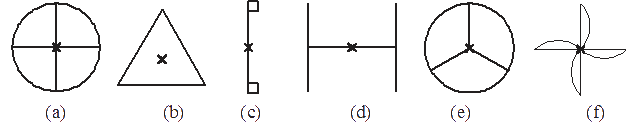# Ex.14.2 Q1 Symmetry - NCERT Maths Class 7

Go back to  'Ex.14.2'

## Question

Which of the following figures have rotational symmetry of order more than $$1$$:Video Solution
Symmetry
Ex 14.2 | Question 1

## Text Solution

What is known?

Figures

What is unknown?

The figures have rotational symmetry of order more than $$1$$.

Reasoning:

To solve this question, remember the concept of rotational symmetry. Rotational symmetry is when an object is rotated around a center point (turned) a number of degrees and the object appears the same. The order of symmetry is the number of positions the object looks the same in a $$360^\circ$$ rotation.

Steps:

Rotational symmetry of order more than one are: (a), (b), (c), (d), (e) and (f)  because in these figures, in a complete turn more than one number of times an object looks exactly the same.

Learn from the best math teachers and top your exams

• Live one on one classroom and doubt clearing
• Practice worksheets in and after class for conceptual clarity
• Personalized curriculum to keep up with school